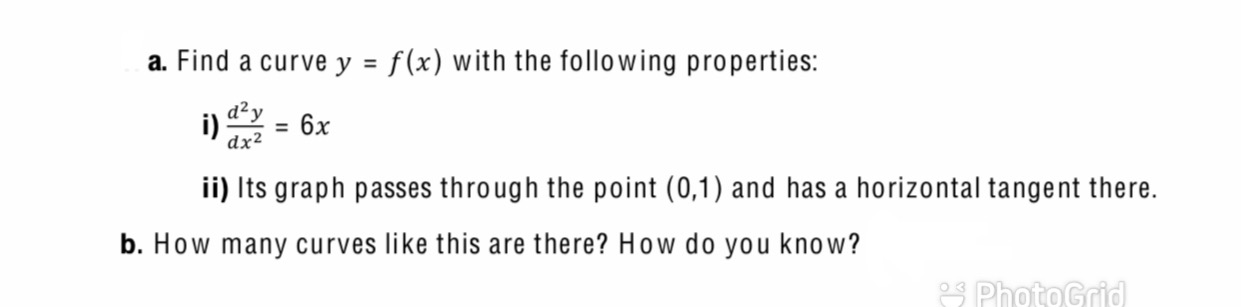# a. Find a curve y = f(x) with the following properties: i) = 6x dx² ii) Its graph passes through the point (0,1) and has a horizontal tangent there. . How many curves like this are there? How do you know?

Questionhelp_outlineImage Transcriptionclosea. Find a curve y = f(x) with the following properties: i) = 6x dx² ii) Its graph passes through the point (0,1) and has a horizontal tangent there. . How many curves like this are there? How do you know? fullscreen

### Want to see this answer and more?

Experts are waiting 24/7 to provide step-by-step solutions in as fast as 30 minutes!*

*Response times may vary by subject and question complexity. Median response time is 34 minutes for paid subscribers and may be longer for promotional offers.
Tagged in
Math
Calculus

### Other